# CnCNet Forums

Search In
• More options...
Find results that contain...
Find results in...

## Recommended Posts

Dear pupils of CnCNet,

From my physics studies I know the formula to calculate velocity:

```v = delta x / delta t
```

Where x is the distance traveled and t the time in which the distance was traveled.

So, say I ran 600 meters in 1.5 minutes (= 90 seconds). My mean velocity would have been:

```v = 600 m / 90 s
v = 6.67 m/s (= 24 km/h)
```

Which makes perfect sense.

Now comes the interesting part. Say I wanted to calculate my acceleration, the formula would be:

```a = delta v / delta t
```

Where v is the difference in velocity and t the time in which said difference was attained.

So, say it takes me 5 seconds to reach my final speed of 6.67 m/s, my acceleration would be:

```a = (6.67 m/s) / (5 s)
a = 1.33 m/(s^2)
```

What the fuck does this tell me? My acceleration is 1.33 m/(s²), cool, so now, what can I make out of it?

I mean I know what a m² (square meter) is. But what the fuck is an s² (square second)? I just can't make anything out of it.

Thanks,

SiRaLeX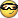##### Share on other sites

I don't know where you live, but in my country it's a basic school knowledge. just draw a function of y(x2) and it will explain you a lot.

##### Share on other sites

I don't know where you live, but in my country it's a basic school knowledge. just draw a function of y(x2) and it will explain you a lot.

I live in Germany. Where do you live?

What do you mean with y(x²)? We're talking about 1.33 m/(s²), I don't really see how y(x²) is applicable here?

##### Share on other sites

in Belarus.

ok, let's look at this from another spot

m/s2 = (m/s)/s

that means how much you speed will increase every second

in your case it will increase by 1.33 m/s every second

just don't try to imagine square seconds, math is an abstract science

##### Share on other sites

Well, to be quite frank, it doesn't make any sense to me. Can you help me draw that graph?!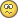This is the only thing I managed to get done, which makes sense to me (see attachment), it's about velocity not acceleration.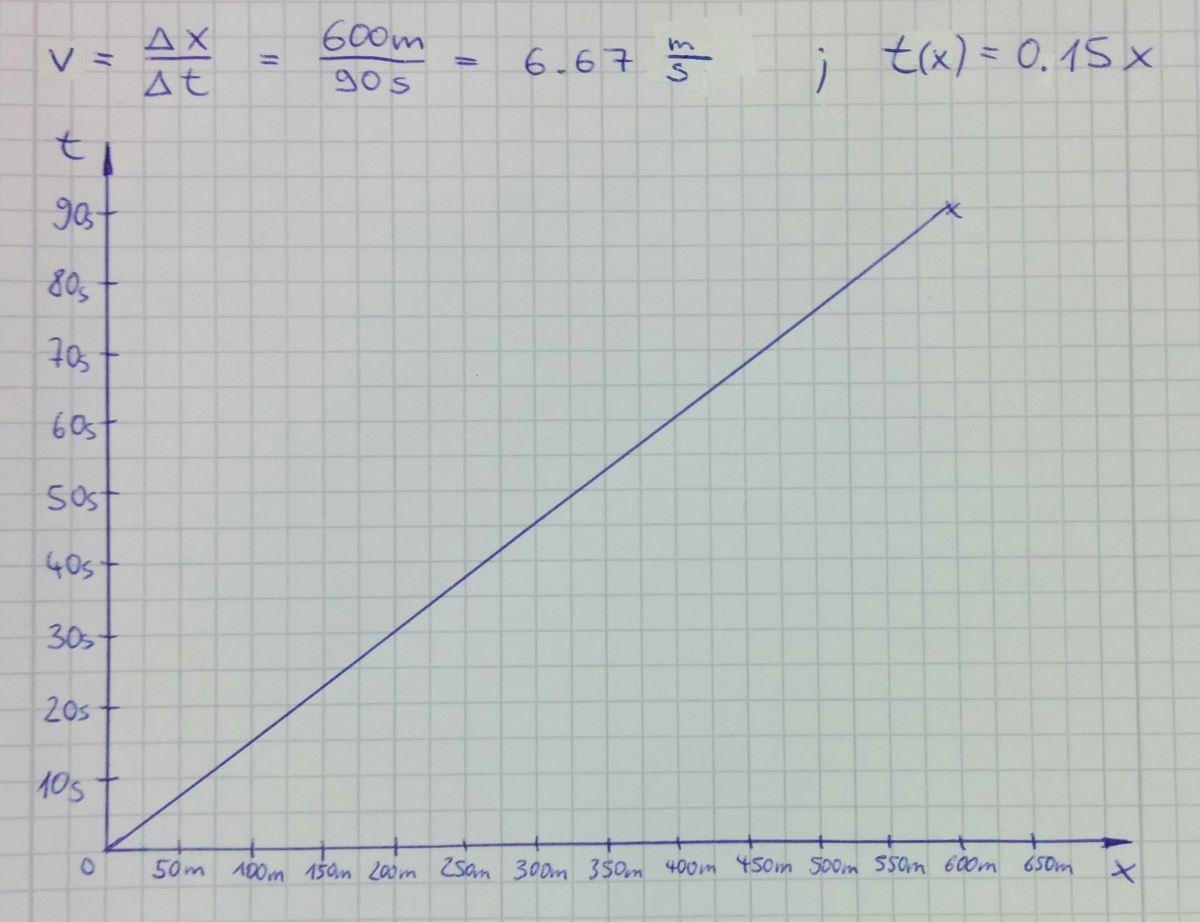##### Share on other sites

Acceleration is the rate of change of velocity. A square Second is just a sort of abstract feature that comes out of what acceleration is, theres no real physical way to explain it other than that.

Try understanding what this unit is: m-3 kg-1 s4 A2. (That particular unit is the permittivity of free space, mostly involved with electromagnetism)

What you will find a lot in Physics is constants and variables with units that from an individual view, make zero sense, but are derived from unit cancelling, or from calculation (like how Acceleration comes to be ms-2)

But your calculation of acceleration is fine (its constant so its not complicated)

using x,v,a,t (distance, speed, acceleration, time) v = dx/dt, and a = dv/dt. Your number 1.33 tells you in one second your speed increases by 1.33ms-1 and that you move 1.33m

##### Share on other sites

Acceleration is the rate of change of velocity.

That's correct! Thanks so much for your post - I'll try to understand it.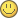In the meantime I drew the acceleration graph (acceleration from 0 m/s to 6.67 m/s in 5 seconds). I still don't have much of an idea how to draw the s² graph though.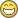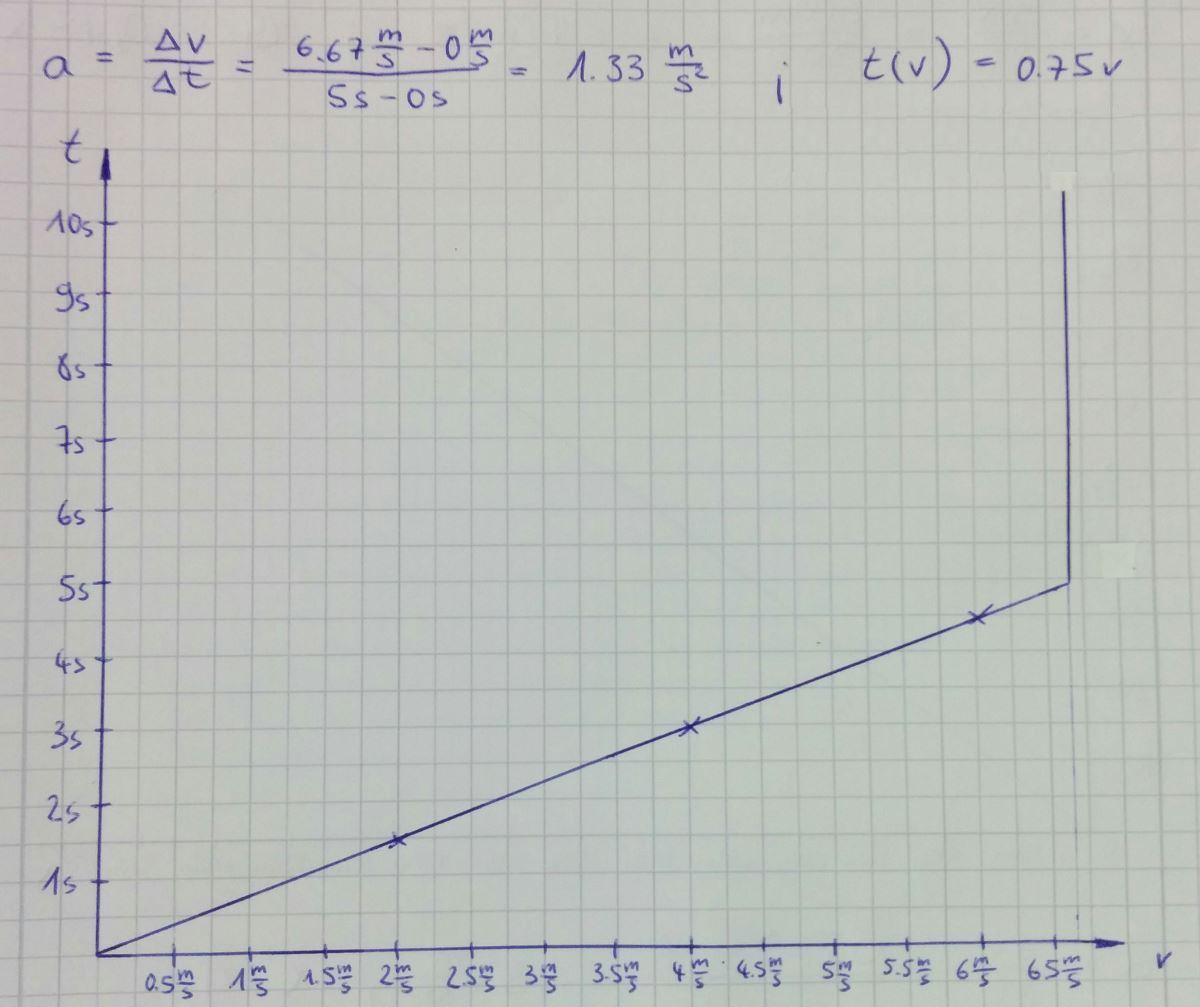##### Share on other sites

you don't plot vs t2 you plot x against t and v against t. The acceleration becomes obvious in the v against t because its a straight line, and the gradient (dv/dt) is your acceleration.

In fact plotting against t2 is harder because then the graph becomes non-linear, and thats just pointless extra work.

##### Share on other sites

you don't plot vs t2 you plot x against t and v against t. The acceleration becomes obvious in the v against t because its a straight line, and the gradient (dv/dt) is your acceleration.

In fact plotting against t2 is harder because then the graph becomes non-linear, and thats just pointless extra work.

ahh, you're right, that explanation is much simplier. I just used to make that kind of things in matlab

##### Share on other sites

He is beating me to it every time.

Just listen to Jacko##### Share on other sites

So are my drawings correct? Should I perhaps switch the axes? Drawing a graph with t² on one side is pointless?

You guys are very knowledgeable, there's no doubt about it!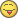##### Share on other sites

When you have troubles with Chemistry, ask me.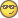If we know the correct questions, we can give you the right answers.

One might shout, that both graphs are incorrect. But the first one is depending on what the exact question was.

I remember that we had to make retarded graphs first for "learning" process. Then we had to discard and start over. Such lovely times.

##### Share on other sites

Man, stop trying to make us do your homework. This is, again, bloody simple.

"m/s" is velocity.

"m/s²" is "(m/s)/s". In other words, velocity per second. Also known as, acceleration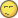##### Share on other sites

Man, stop trying to make us do your homework. This is, again, bloody simple.

"m/s" is velocity.

"m/s²" is "(m/s)/s". In other words, velocity per second. Also known as, accelerationOh, really? Didn't know what velocity and acceleration was. That's why I was able to calculate them.  :roll:

You're very smart aren't you? Took you a while to come up with that, though and no, it's not homework. It's a bogus example asked to understand the concept of s², whereas the better way to put it is indeed (m/s)/s as s² on itself just doesn't make any sense (I believe) - I know that now. Milkey Wilkey and Jacko already explained that very well. When I search in Google for "square second" the very first results it gives me are "meter per second squared".

Just an example I made up, not homework or do you think dividing 6 by 1.5 is homework I have to do? Not even related to homework. Homework would be about Newtonian dynamics, if there was any homework.##### Share on other sites

Homework would be about Newtonian dynamics, if there was any homework.Wouldn't that be exactly that part of high-school Newton that covers acceleration?

##### Share on other sites

Wouldn't that be exactly that part of high-school Newton that covers acceleration?

Don't think so: https://en.wikipedia.org/wiki/Newtonian_dynamics

##### Share on other sites

In physics, the Newtonian dynamics is understood as the dynamics of a particle or a small body according to Newton's laws of motion.

Typically, the Newtonian dynamics occurs in a three-dimensional Euclidean space, which is flat. However, in mathematics Newton's laws of motion can be generalized to multidimensional and curved spaces. Often the term Newtonian dynamics is narrowed to Newton's second law ma = F.

The vector sum of the external forces F on an object is equal to the mass m of that object multiplied by the acceleration vector a of the object: F = ma.

##### Share on other sites

The vector sum of the external forces F on an object is equal to the mass m of that object multiplied by the acceleration vector a of the object: F = ma.

There's your m/s²##### Share on other sites

Hell yeah,

kg*m/s²

Or in other words.

N

When you put some force into things you go...

...

... Nnnnnnnnnnnnnnnnnnnnnn!!!!!

##### Share on other sites

You're right!

1 N = 1 kg * m/s²

I prefer not to know the definition of a Newton but rather that Newton is supposed to come out of a formula I'm inserting values into.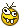##### Share on other sites

Alex, if you want to understand this on a simple level rather than take all the time to decipher various wiki's and such, check this out:

Now go to the part where it says "What are acceleration vs. time graphs"

Note: You can also check out the "What are velocity vs. time graphs"

I'm pretty sure this will cover your questions.

##### Share on other sites

Thank you so much, PreP!##### Share on other sites

Haha, can't touch this!## Create an account

Register a new account